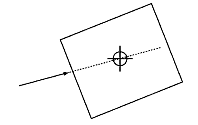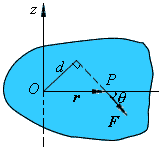# 7．刚体动力学

## 物理知识

F＝ma＝mdvC/dtM＝Fd

Mr×F         （公式1）

M＝Iα＝Idω/dt

Mdt＝I(ωω0)                （公式2）

## 在Stun2Dphyisics中的实现

### 1．在Body类中添加转动惯量

///
/// Body的转动惯量。
/// 2D空间中的转动惯量是一个标量，表示body绕质心转动的难易程度。
/// 不同形状的物体转动惯量也不同。对于诸如圆、矩形之类的基本形状来说，有公式
/// 可以根据形状属性（圆半径或矩形的长宽）计算转动惯量。
/// 若Body不是基本形状，通常也可以使用接近的基本形状进行近似模拟。
/// 还可以使用更高级的算法计算非基本形状的转动惯量。
///
/// M转动惯量不能小于等于0
public float MomentOfInertia
{
get { return momentOfInertia; }
set
{
if (value <= 0)
throw new ArgumentException("转动惯量不能为小于等于0", "value");

momentOfInertia = value;

if (isStatic)
inverseMomentOfInertia = 0;
else
inverseMomentOfInertia = 1f / value;
}
}

///
/// 转动惯量的倒数(1/)
///
public float InverseMomentOfInertia
{
get { return inverseMomentOfInertia; }
}

### 2．添加工厂类自动创建Body

Body body=new Body();
Body.Mass=1;
// 若Body为质量为1，长宽各为1的矩形，根据以上物理知识，它的转动惯量为
// 1*(12+12)/12=1/6
Body. MomentOfInertia=1/6; 

namespace Stun2DPhysics4SL.Factories
{
///
/// 便于创建Body的工厂方法
///
public class BodyFactory
{
private static BodyFactory _instance;

private BodyFactory()
{
}

public static BodyFactory Instance
{
get
{
if (_instance == null)
{
_instance = new BodyFactory();
}
return _instance;
}
}

#region 矩形
///
/// 创建一个矩形Body。
///
/// 物理引擎。
/// 矩形宽。
/// 矩形高。
/// 质量。
///
public Body CreateRectangleBody(PhysicsSimulator physicsSimulator, float width, float height, float mass)
{
Body body = CreateRectangleBody(width, height, mass);
return body;
}

///
/// 创建一个矩形Body。
///
/// 矩形宽。
/// 矩形高。
/// 质量。
///
public Body CreateRectangleBody(float width, float height, float mass)
{
if (width <= 0)
throw new ArgumentOutOfRangeException("width", "矩形宽必须大于0");

if (height <= 0)
throw new ArgumentOutOfRangeException("height", "矩形高必须大于0");

if (mass <= 0)
throw new ArgumentOutOfRangeException("mass", "质量必须大于0");

Body body = new Body();
body.Mass = mass;

// 设置矩形的转动惯量。
body.MomentOfInertia = mass * (width * width + height * height) / 12;
return body;
}
#endregion

#region 圆形
///
/// 创建一个圆形Body。
///
/// 物理引擎。
/// 圆半径。
/// 质量。
///
public Body CreateCircleBody(PhysicsSimulator physicsSimulator, float radius, float mass)
{
return body;
}

///
/// 创建一个圆形Body。
///
/// 圆形半径。
/// 质量。
///
public Body CreateCircleBody(float radius, float mass)
{

if (mass <= 0)
throw new ArgumentOutOfRangeException("mass", "质量必须大于0");

Body body = new Body();
body.Mass = mass;

// 设置圆形的转动惯量
body.MomentOfInertia = .5f * mass * (float)Math.Pow(radius, 2f);
return body;
}
#endregion
}
}

### 3．添加转动方法

private float torque;     // Body所受合力矩

/// <summary>
/// 在下一次更新时施加在Body上的合力矩。
/// 在每次更新调用后都会清零，因此这个值必须在更新前被调用。
/// 力矩可以看成是力的rotational analog。
/// 这个属性是只读的。
/// </summary>
/// 力矩。
public float Torque { get { return torque; } }

dw = AngularAcceleration * dt;

dw = torque * inverseMomentOfInertia * dt;

#region ApplyForceAtLocalPoint临时变量
private Vector2 diff;
#endregion

/// <summary>
/// 在世界坐标系施加一个力，若这个力不过质点，则会施加力矩使刚体转动。
/// </summary>
/// 力。
/// 世界坐标系中的位置。
public void ApplyForceAtWorldPoint(Vector2 setForce, Vector2 point)
{
Vector2.Subtract(ref point, ref position, out diff);

float _torque = diff.X * setForce.Y - diff.Y * setForce.X;

// 施加力矩
torque += _torque;

// 施加力
force.X += setForce.X;
force.Y += setForce.Y;
}

V1(x1, y1)×V2(x2, y2) = x1y2 – y1x2

/// <summary>
/// 在Body上施加一个力矩。
/// </summary>
/// 力矩。
public void ApplyTorque(float setTorque)
{
torque += setTorque;
}

/// <summary>
/// 清除施加在Body上的力矩。
/// 在每次更新后都要调用这个方法。
/// </summary>
public void ClearTorque()
{
torque = 0;
}

 /// <summary>
/// 施加角冲量。
/// </summary>
/// 角冲量。
public void ApplyAngularImpulse(float setImpulse)
{
AngularVelocity += setImpulse * inverseMomentOfInertia;
}

### 4 . 在PhysicsSimulator.cs中添加清除力矩的方法

body.ClearTorque();

## 在Silverlight中的实现

// Sprite长和宽
protected float width;
protected float height; 

public override UserControl CreateSpriteUC()
{
width = 64;
height = 64;
return new Box2();
}

private void Page_KeyUp(object sender, KeyEventArgs e)
{
// 如果点击1键则在上面一个矩形左上角施加一个向右的力
if (e.Key == Key.D1 )
{
box1.Body.ApplyForceAtWorldPoint(new Vector2(9000, 0), new Vector2(32, 98));
box1.Body.IsStatic = false;
}
// 如果点击2键，则在中间一个矩形上施加一个力矩
if (e.Key == Key.D2 & previousKey != e.Key)
{
box2.Body.ApplyTorque(200000);
}
// 如果点击3键
if (e.Key == Key.D3 & previousKey != e.Key)
{
box3.Body.ApplyAngularImpulse(3400);
}
}

2006 - 2023，推荐分辨率1024*768以上，推荐浏览器Chrome、Edge等现代浏览器，截止2021年12月5日的访问次数：1872万9823 站长邮箱